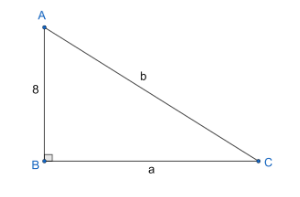Courses
Courses for Kids
Free study material
Free LIVE classes
MoreLIVE
Join Vedantu’s FREE Mastercalss

# In a given triangle ABC, the length of the side AB is given as 8 units. Find the values of ‘a’ and ‘b’ if the value of a + b = 32.Verified
334.5k+ views
Hint: We use the fact that the side opposite to the right angle is the larger side of the triangle. We have given a + b = 32 and we apply pythagoras theorem for the remaining two sides. On applying Pythagora's theorem, we get another linear equation in ‘a’ and ‘b’. Using this new equation and given a + b = 32, we find the values of ‘a’ and ‘b’.

We have given triangle ABC and length of the side AB = 8 units. We need to find the lengths of the remaining sides ‘a’ and ‘b’ using the given condition a + b = 32.
Let us assume a + b = 32 be equation (1).
From the given figure we can see that the given triangle ABC is a right-angled triangle with right angle at B. We know that in a right-angled triangle, the side opposite to the right angle is known as hypotenuse and it is the largest side of the right-angled triangle.
According to the pythagoras theorem, we know that the sum of the squares of the remaining two sides of the right-angled triangle is equal to the square of the hypotenuse.
i.e., $A{{B}^{2}}+B{{C}^{2}}=C{{A}^{2}}$ ---(2).
From the figure, we can see that the value of AB = 8 units, BC = a units and CA = b units.
We substitute these values in equation (2).
${{8}^{2}}+{{a}^{2}}={{b}^{2}}$ .
${{8}^{2}}={{b}^{2}}-{{a}^{2}}$ .
We know that $\left( {{x}^{2}}-{{y}^{2}} \right)=\left( x-y \right)\times \left( x+y \right)$ .
$64=\left( b-a \right)\times \left( b+a \right)$ .
From equation (1),
$64=\left( b-a \right)\times 32$ .
$\left( b-a \right)=\dfrac{64}{32}$ .
$\left( b-a \right)=2$ .
$b=2+a$ ---(3).
We substitute equation (3) in equation (1).
$a+\left( 2+a \right)=32$ .
2a + 2 = 32.
2a = 32 – 2.
2a = 30.
$a=\dfrac{30}{2}$ .
a = 15.
We use the value of ‘a’ in equation (3) to get the value of ‘b’.
b = 2 + 15.
b = 17.
∴ The values of ‘a’ and ‘b’ are 15 and 17.

Note: We used Pythagoras theorem to solve the problem and we should not confuse it with the hypotenuse side. We can see that the value of ‘b’ is greater than the value of ‘a’, which proves our claim that hypotenuse is the largest side in the right-angled triangle.
Last updated date: 22nd Sep 2023
Total views: 334.5k
Views today: 7.34k